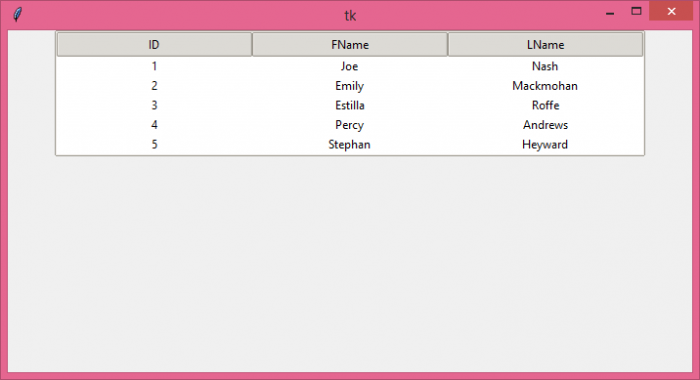# Creating a table look-a-like using Tkinter

A table contains data items in the form of rows and columns. Consider a case of having a table GUI in an application where we can manipulate the data using other Python libraries such as Numpy, Pandas, Matplotlib, etc. Tkinter provides TreeView widget which enable the user to draw the table and insert the data into it. The TreeView widget can be constructed by defining the Treeview(parent, column, **options) constructor.

## Example

# Import the required libraries
from tkinter import *
from tkinter import ttk

# Create an instance of tkinter frame
win = Tk()

# Set the size of the tkinter window
win.geometry("700x350")
s = ttk.Style()
s.theme_use('clam')

tree = ttk.Treeview(win, column=("c1", "c2", "c3"), show='headings', height=5)
tree.column("# 1", anchor=CENTER)
tree.column("# 2", anchor=CENTER)
tree.column("# 3", anchor=CENTER)

# Insert the data in Treeview widget
tree.insert('', 'end', text="1", values=('1', 'Joe', 'Nash'))
tree.insert('', 'end', text="2", values=('2', 'Emily', 'Mackmohan'))
tree.insert('', 'end', text="3", values=('3', 'Estilla', 'Roffe'))
tree.insert('', 'end', text="4", values=('4', 'Percy', 'Andrews'))
tree.insert('', 'end', text="5", values=('5', 'Stephan', 'Heyward'))

tree.pack()

win.mainloop()

## Output

Running the above code will display a window that contains a table with some rows and columns in it.